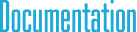#SpatialTrans6< TYPE > Class Template Reference

## Detailed Description

### template<typename TYPE> class cy::SpatialTrans6< TYPE >

6D spatial matrix for coordinate transforms.

This is a special case for SpatialMatrix6 class, where the matrix represents a coordinate transformation. In this case, instead of keeping a full 6x6 matrix values, we can keep a 3x3 matrix for rotation, and a 3D point for translation. This compact representation simplifies some computations, therefore you should use this class instead of SpatialMatrix6 whenever you represent a coordinate transformation. However, for general matrix operations, you have to use SpatialMatrix6.

`#include <cySpatial.h>`

## Public Member Functions

Constructors
SpatialTrans6 ()

SpatialTrans6 (const SpatialTrans6 &mat)

SpatialTrans6 (const Matrix3< TYPE > &_R, const Point3< TYPE > &_r)

Initialization methods
void Set (const Matrix3< TYPE > &_R, const Point3< TYPE > &_r)

void SetIdentity ()

Unary operators
SpatialTrans6 operator- () const

Binary operators
SpatialVector6< TYPE > operator* (const SpatialVector6< TYPE > &p) const

SpatialTrans6 operator* (const SpatialTrans6 &mat) const

SpatialTrans6 operator+ (const SpatialTrans6 &mat) const

SpatialTrans6 operator- (const SpatialTrans6 &mat) const

SpatialTrans6 operator* (TYPE t) const

SpatialTrans6 operator/ (TYPE t) const

Assignment operators
void operator*= (const SpatialTrans6 &mat)

void operator+= (const SpatialTrans6 &mat)

void operator-= (const SpatialTrans6 &mat)

void operator*= (TYPE t)

## Public Attributes

Matrix3< TYPE > R

Point3< TYPE > r

## § R

 Matrix3 R

Rotation matrix.

## § r

 Point3 r

Transformation.